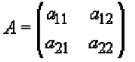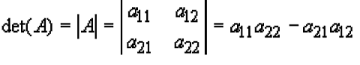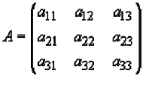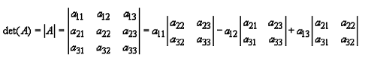Courses

# Calculation of Values of Determinants upto Third Order - Matrices and Determinants, Business Mathema B Com Notes | EduRev

## B Com : Calculation of Values of Determinants upto Third Order - Matrices and Determinants, Business Mathema B Com Notes | EduRev

The document Calculation of Values of Determinants upto Third Order - Matrices and Determinants, Business Mathema B Com Notes | EduRev is a part of the B Com Course Business Mathematics and Statistics.
All you need of B Com at this link: B Com

### What is Matrix Determinant?

The determinant is a scalar value assigned to a square matrix. Matrices which are not square do not have a determinant. The determinant of a (1x1) matrix is just its value, e.g |4| = 4 Straight lines are used instead of square brackets to denote the determinant. The determinants of (2x2) and (3x3) matrices are straightforward to calculate

A determinant is a property that is unique to matrices. In a way, a determinant is like a magnitude. It gives you some information about the resultant matrix in a matrix multiplication operation. Not all matrices have determinants. It is your task to determine when a matrix will have a determinant, and when it will not. To denote that you are taking a determinant, you can use either of the notations below
det A = |A|
Notice that the second notation is also used to find magnitudes and absolute values, depending on the system. This further supports the claim that a determinant is like a magnitude

### How to Find Determinant of a 2x2 Matrix

1. Multiply the entry in the first row and first column by the entry in the second row and second column
If we are finding the determinant of the 2x2 matrix A, then calculate a11 x a22
2. Multiply the entry in the first row and second column by the entry in the second row and first column
If we are finding the determinant of the 2x2 matrix A, then calculate a12 x a21
3. Subtract the second value from the first value 2x2 Matrix2x2 Matrix Determinant Formula### How to Find Determinant of a 3x3 Matrix

1. Extend the matrix by writing the first and second columns again on the right side of the third column
2. Multiply the three entries on the diagonal from the first row and first column entry to the third row and third column entry. If we are finding the determinant of the 3x3 matrix B, then calculate b11*b22*b33
3. Repeat this diagonal multiplication for all three diagonals If we are finding the determinant of the 3x3 matrix B, then calculate b12*b23*b31, and b13*b21*b32
5. Multiply the three entries on the diagonal from the first row and third column entry to the third row and first column entry If we are finding the determinant of the 3x3 matrix B, then calculate b13*b22*b31
6. Repeat this diagonal multiplication for all three diagonals If we are finding the determinant of the 3x3 matrix B, then calculate b11*b23*b32, and b12*b21*b33
7. Add these products and subtract the result from the previous total

3x3 Matrix3x3 Matrix Determinant FormulaOffer running on EduRev: Apply code STAYHOME200 to get INR 200 off on our premium plan EduRev Infinity!

122 videos|142 docs

,

,

,

,

,

,

,

,

,

,

,

,

,

,

,

,

,

,

,

,

,

,

,

,

;